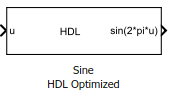# Sine HDL Optimized

Implement fixed-point sine wave by using lookup table approach optimized for HDL code generation

## Library

HDL Coder™ / Lookup Tables

•## Description

The Sine HDL Optimized block implements a fixed-point sine wave by using a lookup table method that exploits quarter-wave symmetry.

For the most efficient HDL implementation, configure the block with an exact power of two as the number of elements. In the Block Parameters dialog box, for Number of data points, specify an integer that is an exact power of two. That is, specify the lookup table data points to be `(2^n)`, where n is an integer. By default, the Number of data points is 64.

When you specify a power of two for the Number of data points, the lookup tables precede a register without reset after HDL code generation. The combination of the lookup table block and register without reset maps efficiently to RAM on the target device.

Depending on your selection of the Output formula parameter, the blocks can output these functions of the input signal:

• sin(2πu)

• cos(2πu)

• exp(iu)

• sin(2πu) and cos(2πu)

Use the Table data type parameter to specify the word length of the fixed-point output data type. The fraction length of the output is the output word length minus 2.

## Data Type Support

The Sine HDL Optimized block accepts signals of these data types:

• Floating point

• Built-in integer

• Fixed point

• Boolean

The output of the block is a fixed-point data type.

## Parameters

Output formula

Select the signal(s) to output.

Number of data points

Specify the number of data points to retrieve from the lookup table. The implementation is most efficient when you specify the lookup table data points to be `(2^n)`, where n is an integer.

Table data type

Specify the table data type. You can specify an expression that evaluates to a data type, for example, `fixdt(1,16,0)`.

Click the buttonto display the Data Type Assistant, which helps you set the table data type.

Show data type assistant

Display the Data Type Assistant. In the Data Type Assistant, you can select the mode to specify the data type.

Mode

Select the mode of data type specification. If you select `Expression`, enter an expression that evaluates to a data type, for example, `fixdt(1,16,0)`.

If you select `Fixed point`, you can use the options in the Data Type Assistant to specify the fixed-point data type. In the `Fixed point` mode, you can choose binary point scaling, and specify the signedness, word length, fraction length, and the data type override setting.

Simulate RAM Delay

Selecting this check box inserts a unit delay without reset. You can simulate this delay in the Simulink modeling environment. When you generate HDL code, a no-reset register is inserted after the block. The combination of lookup table with no-reset register maps to RAM on the target hardware.

## Characteristics

 Data Types Double | Single | Boolean | Base Integer | Fixed-Point Sample Time Inherited from driving block Direct Feedthrough Yes Multidimensional Signals No Variable-Size Signals No Zero-Crossing Detection No Code Generation Yes